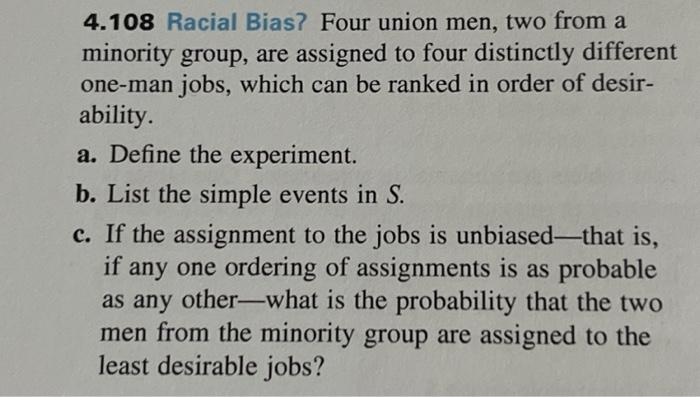Home / Expert Answers / Statistics and Probability / 4-108-racial-bias-four-union-men-two-from-a-minority-group-are-assigned-to-four-distinctly-differ-pa626

# (Solved): 4.108 Racial Bias? Four union men, two from a minority group, are assigned to four distinctly differ ...

4.108 Racial Bias? Four union men, two from a minority group, are assigned to four distinctly different one-man jobs, which can be ranked in order of desir- ability. a. Define the experiment. b. List the simple events in S. c. If the assignment to the jobs is unbiased-that is, if any one ordering of assignments is as probable as any other-what is the probability that the two men from the minority group are assigned to the least desirable jobs?4.108 Racial Bias? Four union men, two from a minority group, are assigned to four distinctly different one-man jobs, which can be ranked in order of desirability. a. Define the experiment. b. List the simple events in . c. If the assignment to the jobs is unbiased-that is, if any one ordering of assignments is as probable as any other-what is the probability that the two men from the minority group are assigned to the least desirable jobs?

We have an Answer from Expert